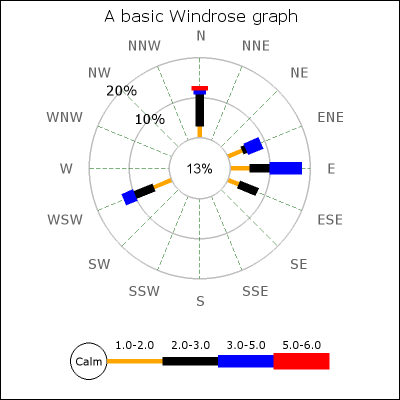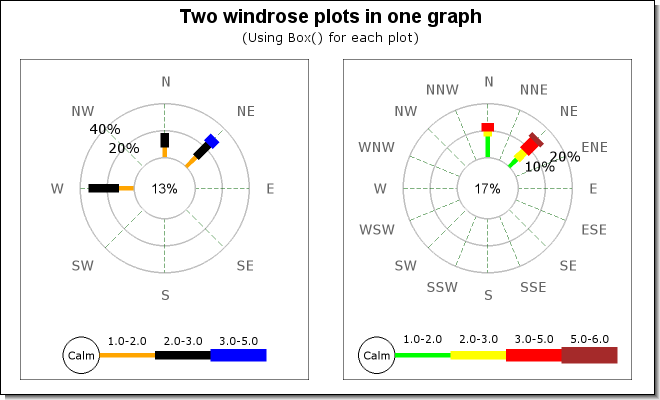## 21.2. Creating and formatting basic Windrose graphs

Creation of windrose graphs first requires the inclusion of the library extension module "`jpgraph_windrose.php`"

The creation of Windrose graphs otherwise follows the traditional steps in the library of creating a graph and then adding one or several windrose plots to the canvas.

1. Create a basic canvas graph as an instance of ```class WindroseGraph```

2. Create an instance of one or several windrose plots as instances of `class WindrosePlot`, set up the scale and appearance and then add them to the graph canvas.

3. Send back the graph to the client with a call to `WindroseGraph::Stroke()`. As usual this can be used to either send back the graph to the client (e.g.browser) or write the graph to a file by specifying a filename as the first argument to `WindroseGraph::Stroke()`.

The example in Figure 21.6 show a windrose graphs using just the default values for all parameters.

Example 21.3. A basic 16 direction windrose graph (`windrose_ex0.php`)

 ```1 2 3 4 5 6 7 8 9 10 11 12 13 14 15 16 17 18 19 20 21 22 23 24 ``` ``` array(5,5,5,8), 1 => array(3,4,1,4), 'WSW' => array(1,5,5,3), 'N' => array(2,3,8,1,1), 15 => array(2,3,5)); // First create a new windrose graph with a title \$graph = new WindroseGraph(400,400); \$graph->title->Set('A basic Windrose graph'); // Create the windrose plot. \$wp = new WindrosePlot(\$data); // Add and send back to browser \$graph->Add(\$wp); \$graph->Stroke(); ?>```

Figure 21.6. A basic 16 direction windrose graph `(windrose_ex0.php)`In the same way as for other graph types one or several Windrose plots can be added and positioned freely in the Windrose graph by specifying the position as either absolute coordinates or as fractions of the width/height of the overall graph.

### Tip

An easier way to position Windrose plots is to use layout classes as described in Section 21.6

Each instance of the `WindrosePlot` is added to the overall graph with a call to the standard method

• `WindroseGraph::Add(\$aObject)`

To avoid that all the plots collide in the middle the positioning and sizing methods as shown in the previous sections should be used.

The following script shows how two plots are displayed in the same graph.

Example 21.4. Adding two windrose plots to the same graph (`windrose_2plots_ex1.php`)

 ```1 2 3 4 5 6 7 8 9 10 11 12 13 14 15 16 17 18 19 20 21 22 23 24 25 26 27 28 29 30 31 32 33 34 35 36 37 38 39 40 41 42 43 ``` ``` array(10,10,13,7), 2 => array(2,8,10), 4 => array(1,12,22), ); \$data2 = array( 4 => array(12,8,2,3), 2 => array(5,4,4,5,2), ); // Create a new small windrose graph \$graph = new WindroseGraph(660,400); \$graph->SetShadow(); \$graph->title->Set('Two windrose plots in one graph'); \$graph->title->SetFont(FF_ARIAL,FS_BOLD,14); \$graph->subtitle->Set('(Using Box() for each plot)'); \$wp = new WindrosePlot(\$data); \$wp->SetType(WINDROSE_TYPE8); \$wp->SetSize(0.42); \$wp->SetPos(0.25,0.55); \$wp->SetBox(); \$wp2 = new WindrosePlot(\$data2); \$wp2->SetType(WINDROSE_TYPE16); \$wp2->SetSize(0.42); \$wp2->SetPos(0.74,0.55); \$wp2->SetBox(); \$wp2->SetRangeColors(array('green','yellow','red','brown')); \$graph->Add(\$wp); \$graph->Add(\$wp2); \$graph->Stroke(); ?>```

Figure 21.7. Adding two windrose plots to the same graph `(windrose_2plots_ex1.php)`### 21.2.1. Specifying the windrose scale

The scale is a property of each plot and is access though the instance variable `WindrosePlot::scale`. The scale consists of the epi-centric circles that radiates from the center of the Windrose plot. The step between each circle can be set manually or it can be done automatically by the library depending on the data values.

(In order to keep the flow of the text this section leaves the full examples to the example section.)

To manual set the scale of the circles the following method is used:

• `WindrosePlotScale::SetStepSize(\$aMax,\$aStepSize=null)`

`\$aMax`, The maximum scale value

`\$aStepSize`, The step between each scale circle

By default the library tries multiple of 5:s and 2:s for a visually esthetic grid spacing.

Other example of methods available to adjust the scale are

• `WindrosePlotScale::SetFont(\$aFontFamily,\$aFontStyle=FS_NORMAL,\$aFontSize=10)`

Specify font for all scale labels apart from the 0-label (in the center)

• `WindrosePlotScale::SetZFont(\$aFontFamily,\$aFontStyle=FS_NORMAL,\$aFontSize=10)`

Specify the font for the 0-label (in the center)

• `WindrosePlotScale::SetFontColor(\$aColor)`

Specify font color for all scale labels apart from the 0-label (in the center)

• `WindrosePlotScale::SetZFontColor(\$aColor)`

Specify the font for the 0-label (in the center)

• `WindrosePlotScale::SetLabelFillColor(\$aBkgColor,\$aBorderColor=false)`

Set the background color for the labels

• `WindrosePlotScale::SetLabelAlign(\$aAlign)`

Set the label text alignment

• `WindrosePlotScale::SetLabelFormat(\$aFmt)`

Set the label format. This is specified as a "`printf()`" format string.

• `WindrosePlotScale::Hide(\$aFlg)`

Hide the scale labels

Examples of how to use these methods are shown in the following sections.

#### Specifying fonts and font colors

Fonts can be specified for both the labels on the scale as well as separately for the label in the center of the plot. The following code snippet shows how to do this (we assume we have already created a windrose plot called "`windplot`")

 ```1 2 3 4 5 6 7 ``` ```// Font and color for scale labels \$windplot->scale->SetFont(FF_VERDANA,FS_NORMAL,10); \$windplot->scale->SetFontColor('navy'); // Font and color for the center (Zero circle) label \$windplot->scale->SetZFont(FF_VERDANA,FS_NORMAL,10); \$windplot->scale->SetZFontColor('navy');```

#### Manually specifying the scale

By default the library automatically determines a scale range and step size taking into account the maximum value of data, size of the plot and the font size specified for the scale labels.

However it is possible to force a manual scale and step size with a call to `Set(\$aMaxValue,\$aStepSize)`. This call sets the maximum scale value as well as the step size. The step size is optional. If the step size is not specified it will be automatically determined.

 ```1 2 3 4 5 ``` ```// Specify both maximum value and a step size \$windplot->scale->Set(40,8); ... // Specify just the maximum value \$windplot->scale->Set(40);```

This feature is also illustrated in Figure 21.25 in the example part of this chapter.

### Note

The automatic scaling tries to first use step sizes as multiples of 5 and then multiples of 2 to achieve a suitable spacing of the scale lines.

### Note

It might be surprising to see that the scale steps may change when the label font is changed. However, this is by design. The reason for this is that there is only a certain amount of space available for the labels between each step. In order for large fonts to fit the space between the grids the step between each circle must be large enough.

#### Specifying the label angle

The labels for the scale is placed along a line radiating from the center of the windrose plots. The angle for the labels can be either manually or automatically determined.

The labels for the scale is placed along one of the sixteen compass directions if you choose automatic positioning of the labels. By default the library tries to choose a direction with as little data around it as possible.

To manually specify the angle a call to `SetAngle(\$aAngle)` with the angle (in degrees) as argument.

The code snippet below shows how to do this

 ```1 2 ``` ```// Show the labels at 45 degrees angle \$windplot->scale->SetAngle(45);```

To specify that the library should do this automatically (which is the default) the angle is specified as the string 'auto' as

 ```1 2 ``` ```// Let the library determine a good angle \$windplot->scale->SetAngle('auto');```

#### Specifying number formats for scale labels

In the same way as for other scales in the library it is possible to specify a "printf()" format string to adjust the appearance of the labels. By default the label format is the same as

 ```1 ``` `\$windplot->scale->SetLabelFormat('%d%%');`

### 21.2.2. Specifying direction labels

With direction labels we mean the names of the compass directions surrounding the windrose plot. By default they are given the English short form for the 16 discrete compass named directions. This can also be localized (described in ??).

#### Specifying fonts and font colors for the axis titles (direction labels)

Fonts for the axis titles are specified with (with the usual arguments)

• `WindrosePlot::SetFont()`

• `WindrosePlot::SetFontColor()`

as the following example shows

 ```1 2 ``` ```\$windplot->SetFont(FF_TIMES,FS_BOLD,12); \$windplot->SetFontColor('darkgreen');```

Which for example could give a Windrose plot as shown in Figure 21.22

#### Setting arbitrary text for data directions

Wen using the free direction windrose plots the default label is the direction for the data in degrees. This is fully customizable by using the following method.

• `WindrosePlot::SetLabels(\$aLabels)`

The input data to this method is an associative array where the keys are the direction and the content is the text to be displayed.

Please note that for regular Windrose plots, i.e. with only compass direction the label can not be changed. This is only available if the type of the windrose plot is `WINDROSE_TYPEFREE`.

The following example specifies a data point at 50 degrees and a text "Point\n#7315" as the label.

### Tip

Labels can contain "\n" which will be interpreted as a line break.

 ```1 2 3 4 5 ``` ```\$data = array( 50 => array(12,12,2) ); \$labels = array( 50 => 'Point #7315' ); ... \$windplot = new WindrosePlot(); \$windplot->SetLabels(\$labels);```

An example of this can be seen in Figure 21.24

#### Adjusting the alignment and margin

There are two basic ways of adjusting the position of the compass labels around the plot:

• Adjusting the margin between the label and the outer plot circle

• Adjusting the anchor point of the labels.

The easiest way to explain this is to first imagine an invisible circle around the plot. The margins specifies how far from the outer scale circle this imaginative circle is placed.

In order to position the labels on that circle around the plot there are several possible ways to select what point of the text should be positioned on the circle. The easiest way is to select the center (both in X and Y directions) of the text. This works well if all labels are roughly the same size. If there are big difference between the smallest and largest label it might be necessary to have a very large margin to avoid the text to "collide" with the plot.

A better way is to determine the closest point of the text to the plot and then make that point lay on the circle. The closets point depends on what compass direction the label is on, for example for the "East" label the closes point is the middle of the left side of the text and for the "West" the closes point is the middle of the right side.

The library supports both way of positioning through the method

• `WindrosePlot::SetLabelPosition(\$aPosition)`

Valid values for \$aPosition are `LBLPOSIITON_CENTER` and `LBLPOSITION_EDGE` .

The following code snippet shows how to specify both margin and position do this:

 ```1 2 ``` ```\$windplot->SetLabelMargin(30); \$windplot->SetLabelPosition(LBLPOSITION_EDGE);```

The red circle in ?? and ?? illustrates the "imaginative" circle on which the labels are placed. The distance from the out most plot circle to the label anchor point is the margin.

 Figure 21.8. Positioning with LBLPOSITION_CENTERFigure 21.9. Positioning with LBLPOSITION_EDGEAn example of this can be seen in Figure 21.24